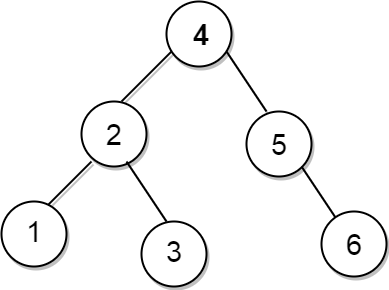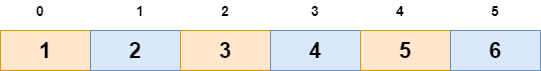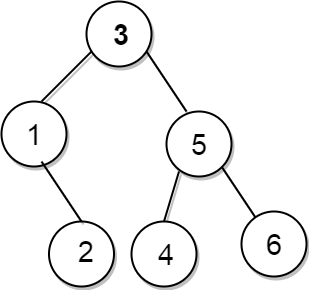# Convert Sorted Array to Balanced BST

## Introduction

In this blog, we will learn how to generate a balanced BST from a sorted array. But before that, we should focus on the term BST or Binary Search Trees. BST are the trees where each node follows the below-mentioned properties:-

• The key value of the left subtree must be smaller than the key value of its parent node.
• The key value of the right subtree must be greater than or equal to that key value of its parent node.

After going through the above definition, you have understood the term BST but now you must be thinking that what does this term Balanced signifying here?

So the balanced tree means that the difference of the height of the left subtree with the height of the right subtree or vice versa cannot be more than one i.e abs(height(left subtree) - height(right subtree)) <= 1. So let’s understand balanced BST with an example:Since we have understood the term Balanced BST clearly. Now let’s move towards the problem statement. So, it goes like this:

Problem: You are given a sorted array in which elements are arranged in ascending order. Convert this sorted array into a Balanced BST.

Suppose we are given a sorted array like this :Then we have to form a balanced output from this array as our output.

Let’s move towards building a solution to this problem.

Since this array is sorted in increasing order then we can divide it into two halves. One half contains smaller elements and the other half contains bigger elements. This is the intuition behind our solution. Let’s discuss it more clearly in steps:

Step 1: Find the middle element of the array and this will be the root of our balanced BST.

Step 2: Then we will do the same for the left half and right half using recursion.

Find the middle of the left half and then this middle will be the left child of our newly created root node.

Find the middle of the right half and then this middle will be the right child of the root node.

Now the question that must be popping in your head is why are we choosing the middle element of the array as the root of our balanced BST?

Good Question! See, when we are making a balanced BST, we want nodes with smaller data to be present on the left side of the root node and nodes with greater elements on the right side of the root node. Another thing we are demanding is that the tree should be balanced. For this, we will try to have equal nodes on both sides of the middle element(root). So after putting these conditions in view, you can quickly answer that the middle element of the array will be a good choice for the root node because since it’s a sorted array all elements on the left side will be smaller than the middle element and on the right of the middle element we will find greater element than the middle element. So this fulfills our first requirement of BST. Since we know that the middle element divides an array into two halves, this fulfills our second requirement. Hence we always prefer to choose the middle element as the root node and recursively build the tree by recursion on the left and right sides of the middle element.

After all these steps, you will get Balanced BST as your output.

C++ code for Balanced BST

``````#include <bits/stdc++.h>
using namespace std;

/* A Binary Tree class */
class Node {
public:
//data members
int data;
Node * left;
Node * right;

//constructor
Node(int data) {
this -> data = data;
left = NULL;
right = NULL;
}
};

/*function that constructs Balanced
BST from a sorted array */
Node * sortedArrToBST(int * arr,
int start, int end) {
/* Base Case */
if (start > end)
return NULL;

// Find the 'mid' index of the array and make it a root node.
int mid = (start + end) / 2;
Node * rootNode = new Node(arr[mid]);

/* Recursively construct the left subtree
and make it left child of root */
rootNode -> left = sortedArrToBST(arr, start,
mid - 1);

/* Recursively construct the right subtree
and make it right child of root */
rootNode -> right = sortedArrToBST(arr, mid + 1, end);

return rootNode;
}

//This function will be printing the formed balanced BST in preOrder.
void preOrder(Node * node) {
if (node == NULL)
return;
cout << node -> data << " ";
//calling this function recursively
preOrder(node -> left);
preOrder(node -> right);
}

int main() {
int arr[] = {
1,
2,
3,
4,
5,
6
};
int n = sizeof(arr) / sizeof(arr);

//calling the function for creating BST
Node * root = sortedArrToBST(arr, 0, n - 1);
cout << "Printing the balanced BST in it's preorder traversal:\n";
//function for printing the tree
preOrder(root);
return 0;
}

Output:
3 1 2 5 4 6``````

The tree we will be getting in output after running this code will look like:-## Complexity Analysis:

Time Complexity: The time Complexity of the above code will be O(N) where N refers to the size of the sorted array.The recurrence relation for the above solution is T(N)  = 2 * T(N / 2) + C , where ‘C’ is constant time. Solving this using the substitution method gives us the overall complexity of O(N).

Space Complexity: The space Complexity of this code will be (log(N)), where ‘N’ is the size of the given array. The recursion call stack will store function calls up to the height of the tree, and the tree is balanced thus height in the worst case will be log(N). Hence the overall space complexity will be O(logN).

Ques 1. What is a Balanced Tree?

Ans 1. The balanced tree means that the difference of the height of the left subtree with the height of the right subtree cannot be more than one.

Ques 2. What is a Preorder Traversal of a Tree?

Ans 2. In a Preorder traversal of a tree root node is printed first, then we print its left child, and then its right child gets printed.

Ques 3. Can I form a balanced BST from an array sorted in decreasing order?

Ans 3. Yes! You can. The algorithm we have discussed here will also be applicable to the array sorted in decreasing order. The only difference is that in the decreasing array you will find smaller elements in the right half instead of the left half.

Ques 4. What is the height of a balanced tree in the worst case?

Ans 4. In the case of balanced trees, the height in the worst case will be O(logN).

## Key takeaways:

In this blog, we discussed the approach for converting a sorted array into a balanced BST. Remember that in a balanced BST, the difference between the height of the left subtree and that of the right subtree should never exceed one. We can do it in O(logN) space complexity here because we are forming a balanced BST here in the case of balanced trees; height never exceeds log(N). Try coding it now on your own here. Do share this blog with your friends. Happy Coding!